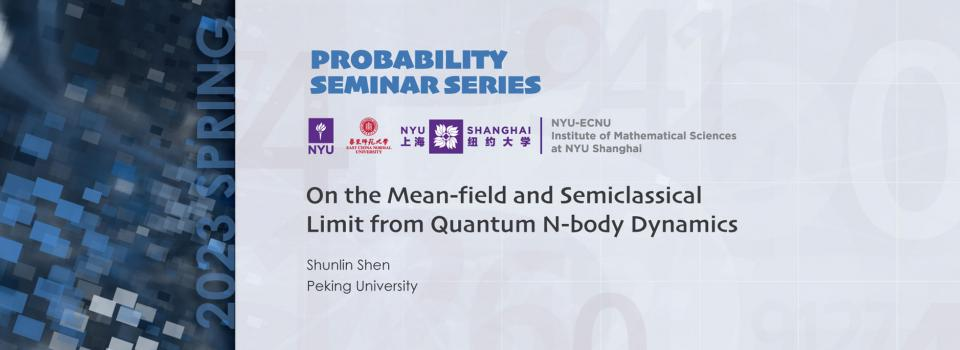# On the Mean-field and Semiclassical Limit from Quantum N-body DynamicsTopic:
On the Mean-field and Semiclassical Limit from Quantum N-body Dynamics
Date & Time:
Thursday, May 18, 2023 - 17:00 to 18:00
Speaker:
Shunlin Shen, Peking University
Location:
W923, NYU Shanghai New Bund Campus & Hosted via Zoom

- RSVP Here -

Abstract:

We study the mean-field and semiclassical limit of the quantum many-body dynamics with a repulsive $\delta$-type potential $N^{3\beta}V(N^{\beta}x)$ and a Coulomb potential, which leads to a macroscopic fluid equation, the Euler-Poisson equation with pressure. We prove quantitative strong convergence of the quantum mass and momentum densities up to the first blow up time of the limiting equation. The main ingredient is a functional inequality on the $\delta$-type potential for the almost optimal case $\beta\in(0,1)$, for which we give an analysis of the singular correlation structure between particles. This talk is based on a joint work with Xuwen Chen and Zhifei Zhang.

Biography:

Shunlin Shen is a postdoctoral fellow at Peking University. He obtained a Ph.D in 2022 at Peking University. His research interests lie in partial differential equations and mathematical physics, especially quantum mean-field limit, quantum many-body problems.

Seminar by the NYU-ECNU Institute of Mathematical Sciences at NYU Shanghai